info@biomedres.us   +1 (502) 904-2126   One Westbrook Corporate Center, Suite 300, Westchester, IL 60154, USA   Site Map

Impact Factor : 0.548

# Growth of Phytoplankton Volume 13 - Issue 2

Udriste C* and Tevy I

• Department of Mathematics-Informatics, Faculty of Applied Sciences, Romania

Received: December 21, 2018;   Published: January 16, 2019

*Corresponding author: Udriste C, Department of Mathematics-Informatics, Faculty of Applied Sciences, Splaiul Independentei 313, Bucharest, 060042, Centenary of Romanian Great Union 1818-2018, Romania

DOI: 10.26717/BJSTR.2019.13.002360

## Abstract

A central problem in biological dynamical systems is to determine the boundaries of evolution. Although this is a general problem, we prefer to give solutions for growth of the phytoplankton. AMS Mathematical Classiftcation: 92D40, 35B36, 35Q92, 37N25.

Keywords: Phytoplankton Dynamical System; Phytoplankton Sub-strate; Phytoplankton Biomass; Intracellular Nutrient Per Biomasss

## Introduction

### Phytoplankton Dynamical System

In the paper of Bernard - Gouze  one analyse a model of phytoplankton growth based on the dynamical systemwhere x1 means the substrate, x2 is the phytoplankton biomass and x3 is the intracellular nutrient per biomass, with the physical domain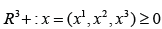The previous dynΣamical system is non cooperative and has the equilibrium pointWe introduce the phytoplankton vector fieldof components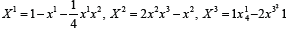and the maximal field line x = x(t, x0), t ∈ I, which satisfies the initial condition x(t0, x0) = x0. In order to find bounds for substrate, biomass, and intracellular nutrient per biomass, we use the techniques of optimization developed in our papers [2-6].

### Bounds for Phytoplankton Substrate

We use the following problem: ftnd max f (x1, x2, x3) = x1 with the restriction x=x(t,x0). We set the critical point condition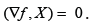. In this caseIt follows the relation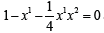The convenient solution (critical point)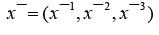must beThe sufficient condition Hessreduces to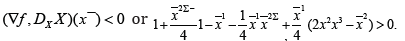Since at the critical point we havethe condition goes to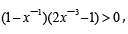and the convenient condition is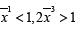. Theorem 2.1. Suppose that on a evolution line (field line) it exists a point x¯ at which we have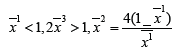Then the phytoplankton substrate has an upper bound at this point.

### Bounds for Phytoplankton Biomass

Let us use the problem: ftnd max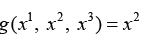subject to x=x(t,x0).

Growth of phytoplankton: The critical point condition is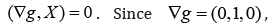it follows the relationThe convenient solution (critical point)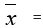The sufficient condition Hess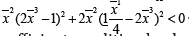Since at the critical point we have , the sufficient condition leads toTheorem: Suppose that on a evolution line (field line) it exists a point at which we havethen the x2(t) component of the corresponding field line has an upper bound at this point.

In the direct alternative, we build the composite function g(x(t, x0)). The conditionreduces to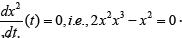the convenient solution isThe condition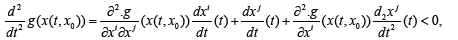becomesReplacingwe find x2 > 0, x1 − 2 < 0. The same result is obtained as in the previous method.

## Bounds for Intracellular Nutrient Per Biomass

Now the helping problem is: compute maxwith the restrictionThe critical point condition isSince Δh = ∇h = (0,0,1), it follows the relation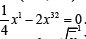The critical pointThe sufficient condition Hess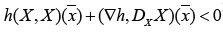reduces to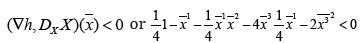Since at the critical point we havethe condition goes toTheorem: Suppose that on a evolution line (field line) it exists a point x at which we haveThen the intracellular nutrient per biomass has an upper bound at this point.